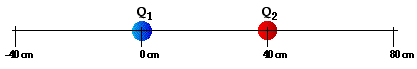# Electricity: Static Electricity

## Static Electricity: Audio Guided Solution

#### Problem 27:

Two charges are separated by a distance of 40 cm. Q1 has a charge of -4.5 nC and Q2 has a charge of +9.1 nC. (GIVEN: 1 C = 109 nC.)Determine the location where the net electric field is 0 N/C. Express your answer as an x-coordinate location (in cm), with Q1 being located at x=0 cm and Q2 being located at x = +40 cm (as shown in the diagram).

## Habits of an Effective Problem Solver

• Read the problem carefully and develop a mental picture of the physical situation. If necessary, sketch a simple diagram of the physical situation to help you visualize it.
• Identify the known and unknown quantities; record them in an organized manner. A diagram is a great place to record such information. Equate given values to the symbols used to represent the corresponding quantity - e.g., Q1 = 2.4 μC; Q2 = 3.8 μC; d = 1.8 m; Felect = ???.
• Use physics formulas and conceptual reasoning to plot a strategy for solving for the unknown quantity.
• Identify the appropriate formula(s) to use.
• Perform substitutions and algebraic manipulations in order to solve for the unknown quantity.